# Ring with divided powers

Letbe a commutative ring with unit, and letbe an augmented-algebra, i.e. there is given a homomorphism of-algebras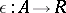. A divided power structure on(or, more precisely, on the augmentation ideal) is a sequence of mappings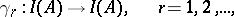such that

1)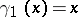;

2)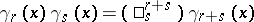;

3)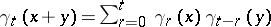;

4);

5);

where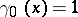in 3) and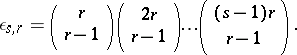In caseis a graded commutative algebra overwith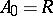, these requirements are augmented as follows (and changed slightly):

6)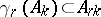,

with 5) replaced by

5')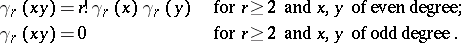Given an-module, an algebra with divided powers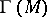is constructed as follows. It is generated (as an-algebra) by symbols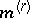,,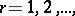and between these symbols the following relations are imposed: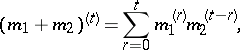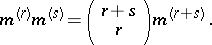Thissatisfies 1)–5). The augmentation sendsto(). If one assigns tothe degree, a graded commutative algebra is obtained with,which satisfies 1)–4), 5'), 6).

Ifis a-algebra, divided powers can always be defined as. The relations 1)–5) can be understood as a way of writing down the interrelations between such "divided powers" (such as the one resulting from the binomial theorem) without having to use division by integers.

A divided power sequence in a co-algebra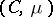is a sequence of elements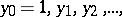satisfying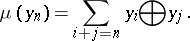Divided power sequences are used in the theories of Hopf algebras and formal groups (cf. Formal group; Hopf algebra), [a1][a3]. Rings with divided powers occur in algebraic topology (where they provide a natural setting for power cohomology operations), [a4], [a5], and the theory of formal groups [a3], [a2].

How to Cite This Entry:
Ring with divided powers. Encyclopedia of Mathematics. URL: http://encyclopediaofmath.org/index.php?title=Ring_with_divided_powers&oldid=16426